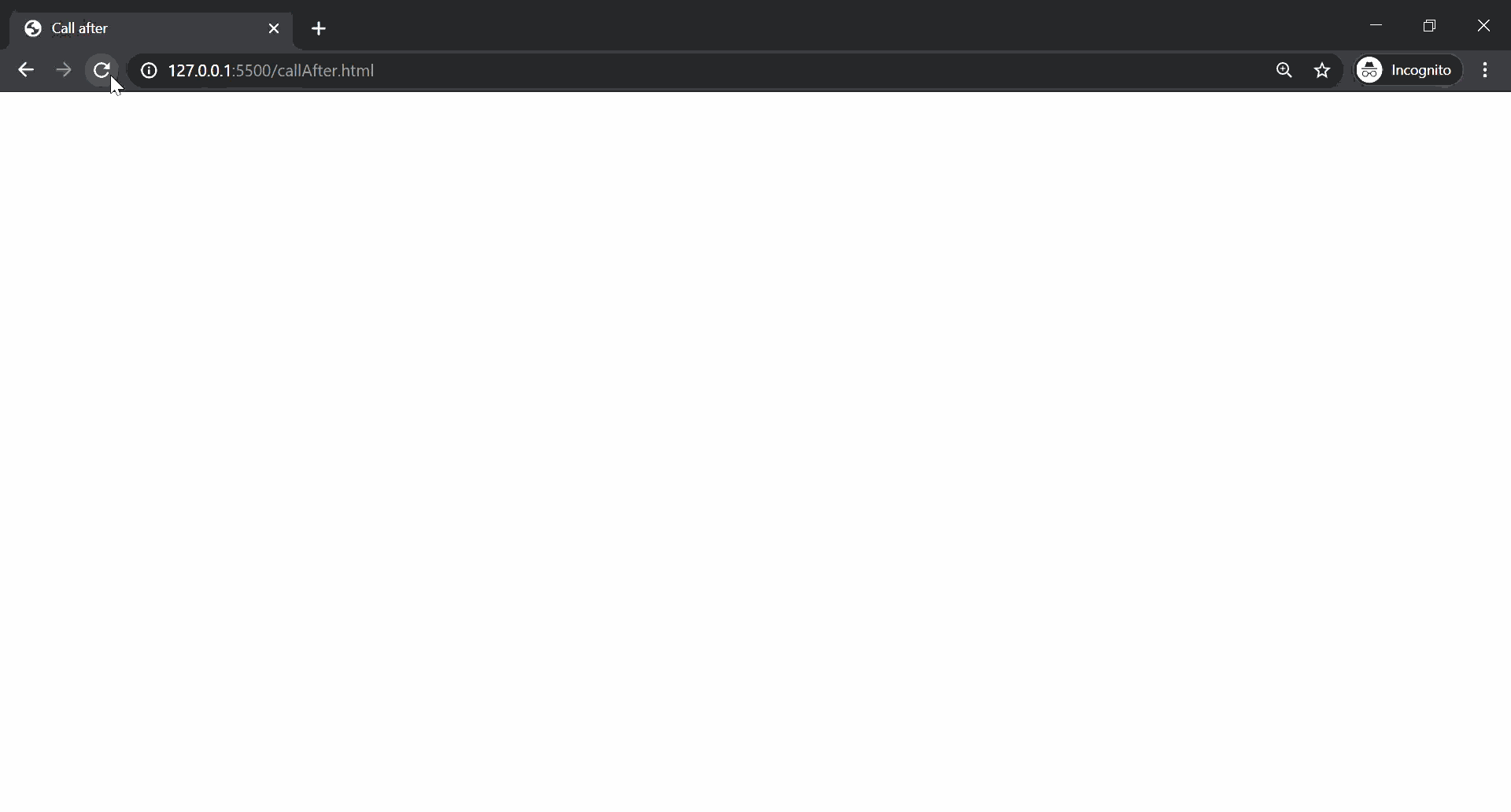# How to implement a function that enable another function after specified time using JavaScript ?

• Last Updated : 12 Mar, 2021

Let us assume that we have been given a function add() which takes in two parameters a and b, and returns their sum. We are supposed to implement a function that should be able to call any function after the given delay amount of time. This can be done with the approaches as given below:

Approach 1: Creating a function that takes in the amount of delay, the function to be called, and the parameters to be given.

In this approach, we will pass the add() function along with its parameters to the callAfter() we have created. The setTimeout() method is used to call the add() function from inside the callAfter() method after the given delay amount of time. The parameters are also passed to the function.

Example:

## HTML

 ` ` `<``html``> ` ` `  `<``body``> ` `    ``<``script``> ` `        ``function callAfter(delay, myFunct, ` `            ``...params) { ` `            ``setTimeout(() => { ` `                ``myFunct(...params); ` `            ``}, delay); ` `        ``} ` ` `  `        ``function add(a, b) { ` `            ``alert("The sum is : " + (a + b)); ` `        ``} ` ` `  `        ``callAfter(2000, add, 4, 7); ` `    `` ` ` ` ` `  ` `

Approach 2: Using an anonymous function.

In some cases, you may want to pass a function with arguments, but not want it to be called until the callback is invoked. In this case, you could wrap it in an anonymous function.

Example:

## HTML

 ` ` `<``html``> ` ` `  `<``body``> ` `    ``<``script``> ` `        ``function callAfter(delay, funct) { ` `            ``setTimeout(() => { ` `                ``funct(); ` `            ``}, delay); ` `        ``} ` ` `  `        ``function add(a, b) { ` `            ``alert("The sum is : " + (a + b)); ` `        ``} ` ` `  `        ``callAfter(2000, function() { ` `            ``add(4, 7); ` `        ``}); ` `    `` ` ` ` ` `  ` `

Approach 3: Using a Prototype in JavaScript: In this approach, we can add a method to the function constructor’s prototype object. This will allow us to use the callAfter() with any function.

Syntax:

`functionName.callAfter(delay, param1, param2, ....);`

Example:

## HTML

 ` ` `<``html``> ` ` `  `<``head``> ` `    ``<``title``>Call after ` ` ` ` `  `<``body``> ` `    ``<``script``> ` `        ``Function.prototype.callAfter = ` `            ``function(delay, ...params) { ` `                ``setTimeout(() => this(...params), delay); ` `            ``}; ` ` `  `        ``function add(a, b) { ` `            ``alert("The sum is : " + (a + b)); ` `        ``} ` ` `  `        ``add.callAfter(2000, 4, 7); ` `    `` ` ` ` ` `  ` `

Output: An alert message is displayed after the specified delay for al the approached mentioned above.My Personal Notes arrow_drop_up
Recommended Articles
Page :